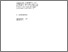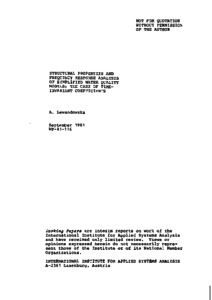Structural Properties and Frequency Response Analysis of Simplified Water Quality Models: The Case of Time-Invariant Coefficients

Lewandowska, A. (1981). Structural Properties and Frequency Response Analysis of Simplified Water Quality Models: The Case of Time-Invariant Coefficients. IIASA Working Paper. IIASA, Laxenburg, Austria: WP-81-116Preview
Text
WP-81-116.pdf

Abstract

This paper deals with the analysis of the structural properties of simplified river water quality models with time-invariant coefficients. The structure of the simplified models should be chosen in such a way as to provide a satisfactory compromise between model accuracy and complexity.

The approach discussed here is based on an analysis of the dynamic properties of the system as well as on the frequency characteristics of the input signals. The analysis of the dynamical properties of the system has been performed for a one-dimensional (in space) time-invariant distributed-parameter model. The unsteady solutions for coupled, partial, differential equations (with two variables: DO and BOD concentrations) with time-invariant coefficients are considered. The model equations are transformed in a special way into diffusion equations, whose solution can be obtained by using the separation of variables method (SVM). As a result, a linear infinite order, ordinary differential equation system, with the same eigenvalues as those of the partial differential equations is obtained. The dynamical properties of the system are characterized here on the basis of a transmittance analysis as well as on the basis of a modal analysis (analysis of eigenfunctions).

The paper concludes with the possibility of choosing a simplified lumped-parameter (finite-order) dynamic or static model of water pollution, which ensures a compromise between accuracy and complexity of the model. It offers, in particular, answers to the following questions: What is the dimensionality of the simplified lumped-parameter model? What is the structure of this model?

The approach is illustrated with results from a case study of the River Cam in eastern England.

Item Type: Monograph (IIASA Working Paper) Resources and Environment Area (REN) IIASA Import 15 Jan 2016 01:49 27 Aug 2021 17:10 http://pure.iiasa.ac.at/1645View Item